GFG App
Open AppBrowser
Continue

# How to Fix: ValueError: Operands could not be broadcast together with shapes?

Broadcasting refers to the ability of NumPy to handle arrays of different shapes during arithmetic operations. Actually, in arrays, Arithmetic operations can be done on corresponding elements of arrays.

• If 2 arrays are of the same shape, then arithmetic operation between 2 arrays can be done smoothly.
• If 2 arrays are of different shapes then element to element operation is not possible. But NumPy makes it possible using the concept of Broadcasting. The smaller array is broadcast to the size of the larger array to make it possible to perform arithmetic operations on them.

Example: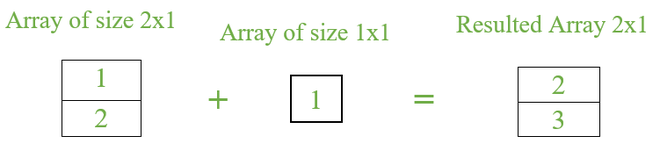Here, a smaller array of size 1×1 is broadcasted to a larger array size as 2×1 where the 2nd row also contains the same element of 1st row i.e. 1. So 1st row=> 1+1=2 & 2nd row => 2+1=3.

If we try to perform an arithmetic operation between 2 arrays of different shapes or different dimensions sometimes NumPy Broadcasting fails. It throws an error like operands could not be broadcast together with shapes. There are some scenarios when broadcasting can occur and when it fails. So if it fails we need to convert the shape of array.

Example: To show where broadcasting fails

## Python3

 `# import necessary packages ` `import` `numpy as np ` ` `  `# create 2 arrays ` `Array1 ``=` `np.arange(``2``).reshape(``1``, ``2``) ` `Array2 ``=` `np.arange(``2``, ``10``).reshape(``3``, ``3``) ` ` `  `# print 2 arrays ` `print``(Array1) ` `print``(Array2) ` ` `  `print``(Array1``+``Array2) `

Output: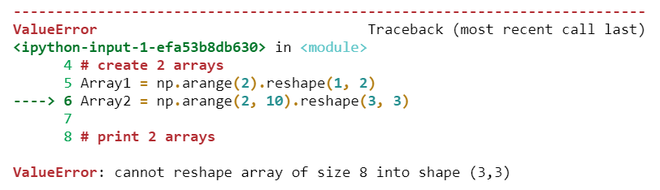Thrown Error

In order to perform broadcasting it internally follow some rules to convert a small-sized array into the shape of a large array. So whenever an error was thrown check the below-mentioned rules to modify the size of the array for successful broadcasting.

### Rule 1:

If both the arrays has same shape or dimensions then no error can be thrown by interpreter. Arithmetic operations can be performed on corresponding elements.

Example: To depict rule 1

## Python3

 `# import necessary packages ` `import` `numpy as np ` ` `  `# create 2 arrays ` `Array1 ``=` `np.arange(``4``).reshape(``2``, ``2``) ` `Array2 ``=` `np.arange(``4``, ``8``).reshape(``2``, ``2``) ` ` `  `# print 2 arrays ` `print``(Array1) ` `print``(Array2) ` ` `  `# addition between 2 arrays ` `print``(``'--Addition--'``) ` `print``(Array1``+``Array2) `

Output: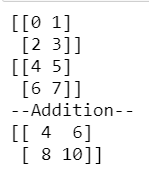#### Explanation

Array1             Array2                                             Result

[[0   1]      +       [[4    5]    =      [[0+4      1+5]       =       [[4     6]

[2   3 ]]              [6    7]]             [2+6      3+7]]                [8    10]]

### Rule 2:

If one array has one dimension as 1 then the adjacent dimensions need to be compared with another array. If those are the same it broadcasts and performs arithmetic operations among them.

Example: To depict rule 2

## Python3

 `# import necessary packages ` `import` `numpy as np ` ` `  `# create 2 arrays ` `Array1 ``=` `np.arange(``2``).reshape(``1``, ``2``) ` `Array2 ``=` `np.arange(``2``, ``8``).reshape(``3``, ``2``) ` ` `  `# print 2 arrays ` `print``(Array1) ` `print``(Array2) ` ` `  `# addition between 2 arrays ` `print``(``'--Addition--'``) ` `print``(Array1``+``Array2) `

Output: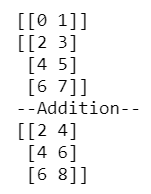Here Array1 has one dimension as value 1 so we need to compare the second dimension, the value of 2 arrays are the same or not. If same, broadcast occurs else fails. We need to convert the size of the array as per the rule. In this example, the values are the same so the addition operation is performed smoothly.

Here Array1 is converted into a 3×2 shape array which is added to Array 2 to give the result.

[[0   1]

[0   1]

[0   1]]

Example: To depict rule 2

## Python3

 `# import necessary packages ` `import` `numpy as np ` ` `  `# create 2 arrays ` `Array1 ``=` `np.arange(``4``).reshape(``2``, ``2``) ` `Array2 ``=` `np.arange(``2``).reshape(``2``, ``1``) ` ` `  `# print 2 arrays ` `print``(Array1) ` `print``(Array2) ` ` `  `# subtraction between 2 arrays ` `print``(``'--Subtraction--'``) ` `print``(Array1``-``Array2) `

Output: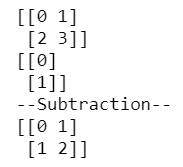### Rule 3:

If the arrays are in the shape of mxn and nxm. In this case, the two arrays are broadcasted into mxm if m>n else nxn if n>m.

Example: To depict Rule 3

## Python3

 `# import necessary packages ` `import` `numpy as np ` ` `  `# create 2 arrays ` `Array1 ``=` `np.arange(``2``).reshape(``2``, ``1``) ` `Array2 ``=` `np.arange(``2``).reshape(``1``, ``2``) ` ` `  `# print 2 arrays ` `print``(Array1) ` `print``(Array2) ` ` `  `# addition between 2 arrays ` `print``(``'--Addition--'``) ` `print``(Array1``+``Array2) `

Output: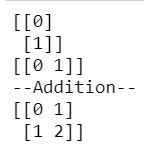#### Explanation

Array1 shape- 2×1

Array2 shape- 1×2    so 2 arrays are broadcasted into shape 2×2.

Arrays are broad casted as follows

Array1     +    Array2       Result

[[0   0]     +   [[0   1]       [[0+0   0+1]

[1   1]]          [0   1]]       [1+0   1+1]]

### Rule 4:

If any of array has its shape 1x1 then no matter about the other array shape it simply broadcast the array of shape 1x1 to shape of another array.

Example: To depict Rule 4

## Python3

 `# import necessary packages ` `import` `numpy as np ` ` `  `# create 2 arrays ` `Array1 ``=` `np.arange(``1``).reshape(``1``, ``1``) ` `Array2 ``=` `np.arange(``2``).reshape(``1``, ``2``) ` ` `  `# print 2 arrays ` `print``(Array1) ` `print``(Array2) ` ` `  `# addition between 2 arrays ` `print``(``'--Addition--'``) ` `print``(Array1``+``Array2) `

Output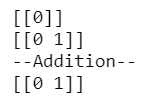The Array1 shape is 1x1 so it is broadcasted to shape of Array2 which is 1x2

Array1    +     Array2   =    Result

[[0  0]]    +    [[0   1]]   =    [[0   1]]

The solution for the above example where broadcasting fails is to simply convert the shape of the Array1 to 1x3  or Array2 shape to 3x2 based on scenario-2.

My Personal Notes arrow_drop_up